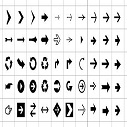# Python Anonymous/Lambda Function

Lambda is another way to define a user define function.It is also called anonymous function which accepts any number of arguments or parameters but returns single object.

Anonymous/Lambda functions are defined using the lambda keyword.

### Syntax:

`lambda arguments : expression`

### Example:

```>>> # lambda a,b : a+b
>>> #to call, to give it a name
30
>>>
```

Lambda function has no name. It returns a function object which is assigned to the identifier add. Then we can call it as a normal function.

### Use of Lambda Function

We use lambda functions when we require a nameless function for a short period of time.

In Python, we generally use it as an argument to a higher-order function (a function that takes in other functions as arguments).

Lambda functions are used along with built-in functions like filter(), map() etc.

### filter() Function :

The filter() function takes in a function and a list as arguments.

The function is called with all the items in the list and a new list is returned which contains only those values corresponding which function evaluates to True.

Below are the execution steps which are performed with filter() function

```Step 1 – Fetch each and every value in iterable
Step 2-  Apply the function
Step 3 – Return Original value for which function is True
```

#### Example:

```>>> list1=list(range(1,10))
>>> list1
[1, 2, 3, 4, 5, 6, 7, 8, 9]
>>> new_list=list(filter(lambda x:x%2==0,list1))
>>> new_list
[2, 4, 6, 8]
>>>
```

### map() function :

The map() function in Python takes in a function and a list.

The function is called with all the items in the list and a new list is returned which contains items returned by that function for each item.

Below are the execution steps which are performed with filter() function :

```Step 1 – Fetch each and every value in iterable
Step 2-  Apply the function
Step 3 – Return evaluated value from function
```

#### Example :

```>>> list1=[x for x in range(1,10)]
>>> list1
[1, 2, 3, 4, 5, 6, 7, 8, 9]
>>> new_list=list(map(lambda x:(x*2),list1))
>>> new_list
[2, 4, 6, 8, 10, 12, 14, 16, 18]
>>>```Ashwini Verma on Apr 17, 2020 at 12:04 am

#### Post Comment

##### WEB TECHNOLOGY##### TypeScript Programmning Language##### New HTML5 APIs##### HTML Arrow -> Range: Decimal 8592-8703. Hex 2190-21FF.##### How To Override One CSS Class With Another×